# （机器学习） – 读书笔记(基础)

1. 基于数据构建统计模型从而对数据进行预测和分析。统计学习可由监督学习、非监督学习、半监督学习和强化学习等组成。
2. 分类任务输出的是离散的值;回归任务输出的连续的值

# 监督学习

## 问题的形式化

$$T=\left\{(\mathbf{x}_1,y_1),\cdots,(\mathbf{x}_i,y_i)\right\}$$

$(\mathbf{x}_i,y_i),\quad i=1,2,\cdots,N$是一个样本点, $\mathbf{x}_i\in\mathcal{X}\subset\textbf{R}^n$是输入的观测值。$y_i\in\mathcal{Y}$是输出的观测值. 在监督学习中训练数据和测试数据是依据联合概率分布$P(X,Y)$独立同分布产生的. 学习过程中,模型使用预测的模型$\hat{P}(Y|X)$或者决策函数$Y=\hat{f}(X)$, 这个条件概率分布描述了输入与输出随机变量之间的映射关系. 衡量模型和真实的数据的标准之一就是预测的输出与实际的标签$y_i$d的误差较小

## 统计学习方法三要素

### 模型

$$\mathcal{F}=\{f|Y=f(X)\}$$

X 和 Y 都是定义在输入空间和输出空间的变量. $\mathcal{F}$是一个由参数向量决定的函数族:

$$\mathcal{F}=\{Y=f_\theta(X),\theta\in\textbf{R}^n\}$$

$$\mathcal{F}=\{P|P(Y|X)\}$$

$$\mathcal{F}=\{P|P_\theta(Y|X),\theta\in\textbf{R}^n\}$$

### 策略

#### 损失函数

1. 0-1 损失函数:

$$L(Y,f(X))=\left\{ \begin{array}{ll} 1, & Y\neq f(X)\\ 0, & Y= f(X) \end{array} \right.$$

1. 平方损失函数:

$$L(Y,f(X))=(Y-f(X))^2$$

1. 绝对损失函数:

$$L(Y,f(X))=|Y-f(X)|$$

1. 对数似然损失函数:

$$L(Y,P(Y|X))=-\log P(Y|X)$$

$$R_\text{emp}(f)=\frac{1}{N}\sum_{i=1}^N L(y_i,f(\mathbf{x}_i))$$

#### 经验风险最小化和结构风险最小化

• 经验风险最小化(empirical risk minimization, ERM)我们需要减小损失:

$$\begin{equation}\min_{f\in\mathcal{F}}\frac{1}{N}\sum_{i=1}^NL(y_i,f(x_i))\label{erm_min}\end{equation}$$

$\mathcal{F}$是假设空间. 在样本容量很小, 经验风险最小化的学习效果未必好, 会产生过拟合的现象.

• 结构风险最小化(structural risk minimization)为了解决过拟合的现象, 则在 ERM 后加上一个正则项

$$R_\text{srm}(f)=\frac{1}{N}\sum_{i=1}^NL(y_i,f(x_i))+\lambda J(f)$$

$J(f)$为模型的复杂度, 是定义在空间$\mathcal{F}$的泛函, 与模型$f$的复杂度成正比. $\lambda\geq0$ 用来权衡经验风险和模型复杂度. 结构风险小的模型需要经验风险和模型复杂度同时小, 这种模型能够很好地对训练时据以及位置的测试数据有很好的预测. 所以要优化的问题就是

$$\begin{equation}\min_{f\in\mathcal{F}}\frac{1}{N}\sum_{i=1}^NL(y_i,f(x_i))+\lambda J(f)\label{srm_min}\end{equation}$$

$\eqref{erm_min}$和$\eqref{srm_min}$都是要解决的最优化问题.

## 模型评估与模型选择

### 训练误差和测试误差

$N$个样本的平均损失:

$$R_\text{emp}(\hat{f})=\frac{1}{N}\sum_{i=1}^NL(y_i,\hat{f}(\mathbf{x}_i))$$

$$e_\text{test}=\frac{1}{N\prime}\sum_{i=1}^{N\prime}L(y_i,\hat{f}(\mathbf{x}_i))$$

### 过拟合和模型选择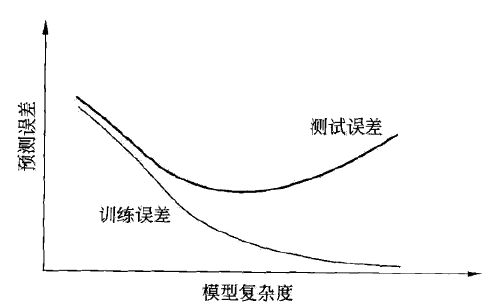### 正则化和交叉验证

#### 正则化

• 回归问题, 损失函数是平方损失, 正则化项是参数向量的$L_2$范数:

$$L(w)=\frac{1}{N}\sum_{i=1}^N(f(\mathbf{x}_i;w)-y_i)^2+\frac{\lambda}{2}||\textbf{w}||^2$$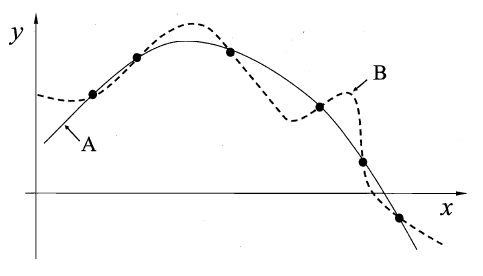#### 交叉验证

• 简单交叉验证

• k折交叉验证(k-ford cross validation)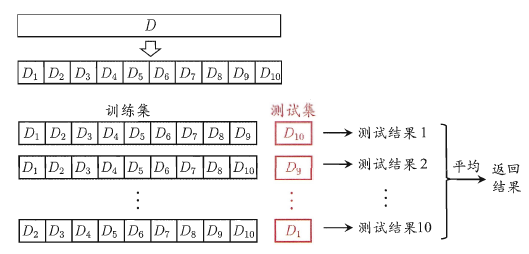• 留一交叉验证

#### 自助法(bootstrapping)

$$\lim_{m\to\infty}(1-\frac{1}{m})^m\to\frac{1}{e}\approx0.368$$

## 性能度量

$$R_\text{exp}(\hat{f})=E_P[L(Y,\hat{f}(X))]=\int_{\mathcal{X}\times\mathcal{Y}}L(y,\hat{f}(\mathbf{x}))P(x,y)d\mathbf{x}dy$$

### 错误率与精度

$$E(\hat{f};D)=\frac{1}{m}\sum_{i=1}^m\mathbb{I}(\hat{f}(\mathbf{x}_i)\neq y_i)$$

\begin{aligned} & \text{acc}(\hat{f};D)=\frac{1}{m}\sum_{i=1}^m\mathbb{I}(\hat{f}(\mathbf{x}_i)=y_i)\\ & = 1-E(\hat{f};D) \end{aligned}

$$E(\hat{f};D)=\int_{x\sim\mathcal{D}}\mathbb{I}(\hat{f}(\mathbf{x})\neq y)p(\mathbf{x})d\mathbf{x}$$

\begin{aligned} &\text{acc}=\int_{x\sim\mathcal{D}}\mathbb{I}(\hat{f}(\mathbf{x})=y)p(\mathbf{x})d\mathbf{x}\\ & = 1-E(\hat{f};D) \end{aligned}

### 查准率、查全率与F1

$$R=\frac{TP}{TP+FN}$$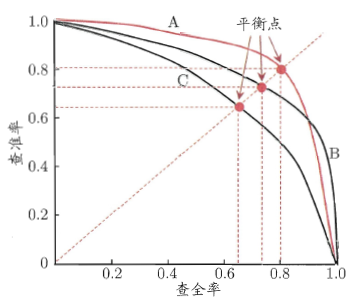$$F1=\frac{2\times P\times R}{P+R}=\frac{2\times{TP}}{\text{样例总数}+TP-TN}$$

$$F_\beta=\frac{(1+\beta^2)\times{P}\times{R}}{(\beta\times{P})+R}$$

$$\text{macro-P}=\frac{1}{n}\sum_{i=1}^n{P_i}$$
$$\text{macro-R}=\frac{1}{n}\sum_{i=1}^n{R_i}$$
$$\text{macro-F1}=\frac{2\times{\text{macro-P}}\times{\text{macro-R}}}{{\text{macro-P}}+{\text{macro-R}}}$$

$$\text{micro-P}=\frac{\overline{TP}}{\overline{TP}+\overline{FP}}$$
$$\text{micro-R}=\frac{\overline{TP}}{\overline{TP}+\overline{FN}}$$
$$\text{micro-F1}=\frac{2\times{\text{micro-P}}\times{\text{micro-R}}}{{\text{micro-P}}+{\text{micro-R}}}$$

### ROC与AUC

$$\text{TPR}=\frac{TP}{TP+FN}$$
$$\text{FPR}=\frac{FP}{TN+FP}$$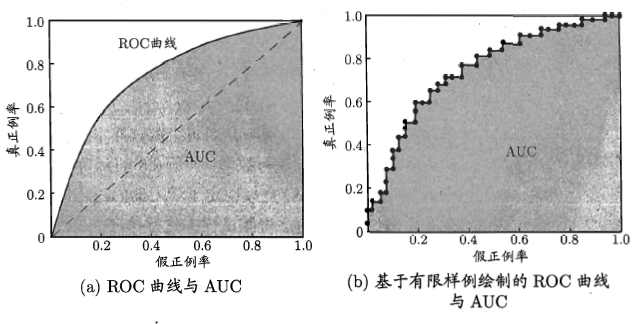$$\text{AUC}=\frac{1}{2}\sum_{i=1}^{m-1}(x_{i+1}-x_i)\cdot{(y_i+y_{i+1})}$$

$$\begin{equation} \mathcal{l}_{\text{rank}}=\frac{1}{m^+m^-}\sum_{x^+\in{D^+}}\sum_{x^-\in{D^-}}(\mathbb{I}(f(x^+)<f(x^-))+\frac{1}{2}\mathbb{i}(f(x^+)=f(x^-)))\label{loss_auc_above} \end{equation}$$
$\mathcal{l}_{\text{rank}}$是对应ROC曲线之上的面积: 若一个正例在ROC曲线之上, 对应坐标为$(x,y)$, 则$x$是排序之前的反例所占的比例, 即假正例率, 因此有:

AUC=1-l_rank

import numpy as np
from sklearn import metrics
y = np.array([1, 1, 2, 2])
scores = np.array([0.1, 0.4, 0.35, 0.8])
fpr, tpr, thresholds = metrics.roc_curve(y, scores, pos_label=2)  # 正例标签为 2
print(fpr,'\n',tpr,'\n',thresholds)
print(metrics.auc(fpr,tpr))  # 计算 AUC
"""

[0.  0.  0.5 0.5 1. ]
[0.  0.5 0.5 1.  1. ]
[1.8  0.8  0.4  0.35 0.1 ]
0.75
"""


$$\text{TPR}=\frac{1}{0+2}=0.5$$
$$\text{FPR}=\frac{0}{2+0}=0$$

$m^+=2\text{,}m^-=2$, 按照公式$\eqref{loss_auc_above}$, $\mathcal{l}_\text{rank}=\frac{1}{4}(1+0+0.5\times{0}+0+0.5\times{0})=\frac{1}{4}$

$$\text{AUC}=1-\frac{1}{4}=\frac{3}{4}=0.75$$

## 比较检验

### 假设检验

$$P(\hat\epsilon;\epsilon) = \begin{pmatrix} m \\ \hat\epsilon\times m \end{pmatrix} \epsilon^{\hat\epsilon\times m}(1-\epsilon)^{m-\hat\epsilon\times m}$$

\begin{aligned} &\overline{\epsilon}=\max\epsilon \\ &\text{s.t.}\quad\sum_{i=\epsilon_0\times{m+1}}^m \begin{pmatrix} m\\ i\\ \end{pmatrix} \epsilon^i(1-\epsilon)^{m-i}<\alpha \end{aligned}

$$\mu=\frac{1}{k}\sum_{i=1}^k\hat{\epsilon_i}$$
$$\sigma^2=\frac{1}{k-1}\sum_{i=1}^k(\hat{\epsilon_i}-\mu)^2$$

$$\tau_t=\frac{\sqrt{k}(\mu-\epsilon_0)}{\sigma}$$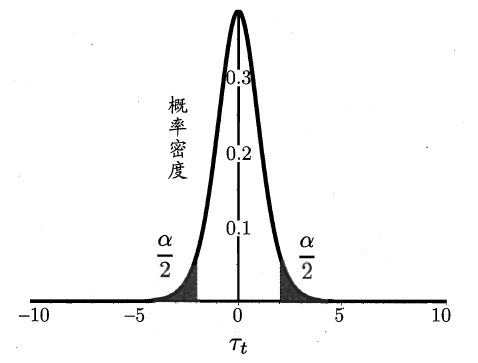## 方差与偏差

• 预测输出

$$\overline{f}(\mathbf{x})=\mathbb{E}_D[f(\mathbf{x};D)]$$

• 使用样本数相同的不同训练加产生的方差: 度量了同样大小的训练集的变动所导致的学习性能的变化，也就是数据扰动所造成的影响:

$$\text{var}(x) = \mathbb{E}_D [(f(x;D)- \bar f (x) )^2]$$

• 噪声(数据集标记和真实标记的方差): 当前任务上任何学习算法所能达到的期望泛化误差的下界，即刻画了学习问题本身的难度:

$$\varepsilon^2 = \mathbb{E}_D [(y_D - y)^2]$$

$$E(f;D) = bias^2(\mathbf{x}) + var(\mathbf{x}) + \varepsilon^2$$

• 若训练不充分, 学习器的拟合能力不强, 训练数据的燃动不足以使学习器产生显著变化, 偏差主导了泛化错误率
• 若过于充足, 学习器拟合能力很强, 训练数据的轻微扰动会导致学习器显著地变化. 若训练数据自身的非全局的特性被学习器学习到, 则发生过拟合.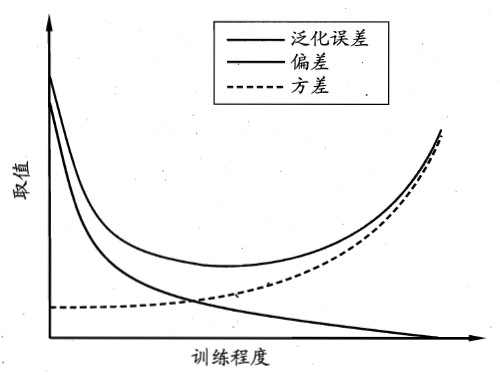# 摘自

1. 《机器学习》周志华 著，2016.1，清华大学出版社
2. 《统计学习方法》李航 著，2012.3，清华大学出版社
3. (CSDN) kingsam_ - AUC的计算方法
4. (wangwlj's Blog)西瓜书《机器学习》学习笔记(2)：比较检验与偏差方差